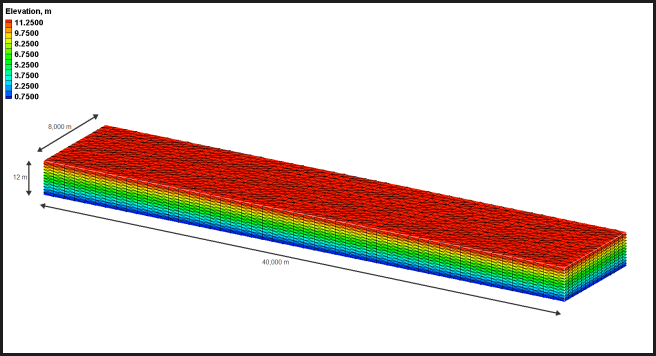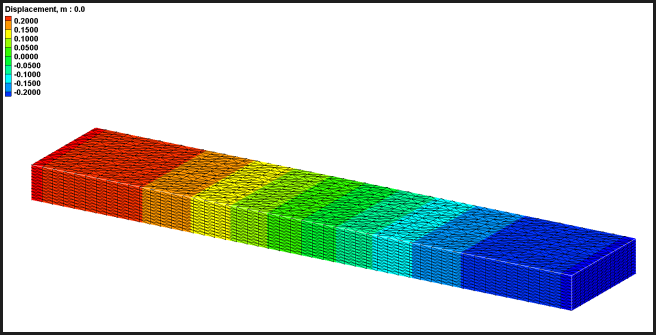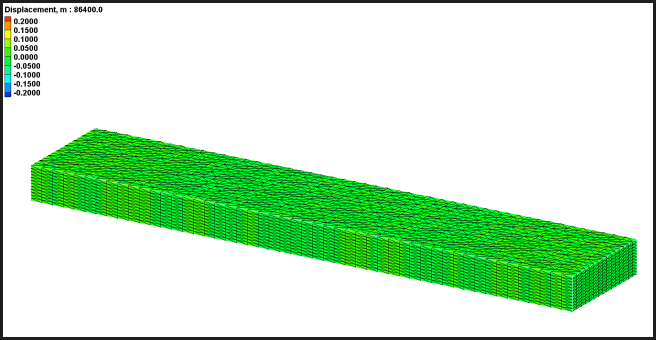## Analytic Mass Conservation

This is an analytic test for the basic tenet of numerical codes utilizing the conservative form of the shallow water equations. AdH is written to be mass conservative and therefore must conserve fluid and constituent mass.

Domain

• Cuboid flume
• 40,000 m length
• 8,000 m width
• 12 m depthInitial Conditions

• +0.25 m water surface elevation at left wall
• -0.25 m water surface elevation at right wall
• 0.035 kg/m3 constituent concentrationBoundary Conditions

• No changes defined over time

Results

• Initial volume of water = 3,840,000,000 m3
• Initial constituent mass = 134,400,000 kg
• Model allowed to slosh for one day
• Final model displacement = 0.0000001 m (~0.0 m)
• Final fluid volume = 3,840,000,000 m3
• Final constituent mass = 134,400,000 kg although some local variations less than 0.002 kg/m3